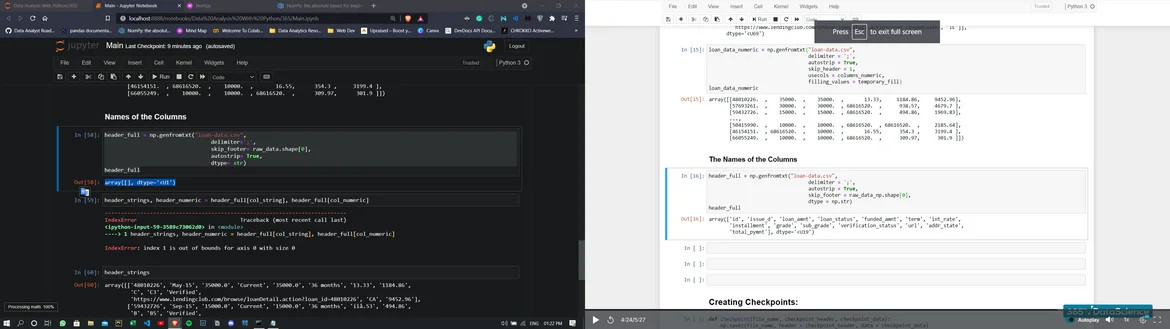01 Mar 2023

Posted on:

02 Nov 2021

1

# index 1 is out of bounds for axis 0 with size 0

While doing the names of the columns part:

``````header_full = np.genfromtxt("loan-data.csv",
delimiter=';',
skip_footer= raw_data.shape,
autostrip= True,
dtype= str)
``````

Generated the output

``````array([], dtype='<U1')
``````

Rather than shown in the videoPosted on:

04 Oct 2022

0

hi ,

to get around this situation do the following

``````raw_data_np = np.genfromtxt("loan-data.csv", delimiter=';', encoding="cp1252",dtype=str)
``````

``````header_full = raw_data_np[0,:]
``````

``````raw_data_np = np.genfromtxt("loan-data.csv", delimiter=';', encoding="cp1252")
``````
Posted on:

04 Oct 2022

0

hi ,

to get around this situation do the following

``````raw_data_np = np.genfromtxt("loan-data.csv", delimiter=';', encoding="cp1252",dtype=str)
``````

``````header_full = raw_data_np[0,:]
``````

``````raw_data_np = np.genfromtxt("loan-data.csv", delimiter=';', encoding="cp1252")
``````
Posted on:

01 Mar 2023

0

I have the same issue, the only close solution I found was this:

names = True,
delimiter = ";",
autostrip = True,
encoding = "cp1252",
dtype = np.str_,
skip_footer = raw_data_np.shape
)